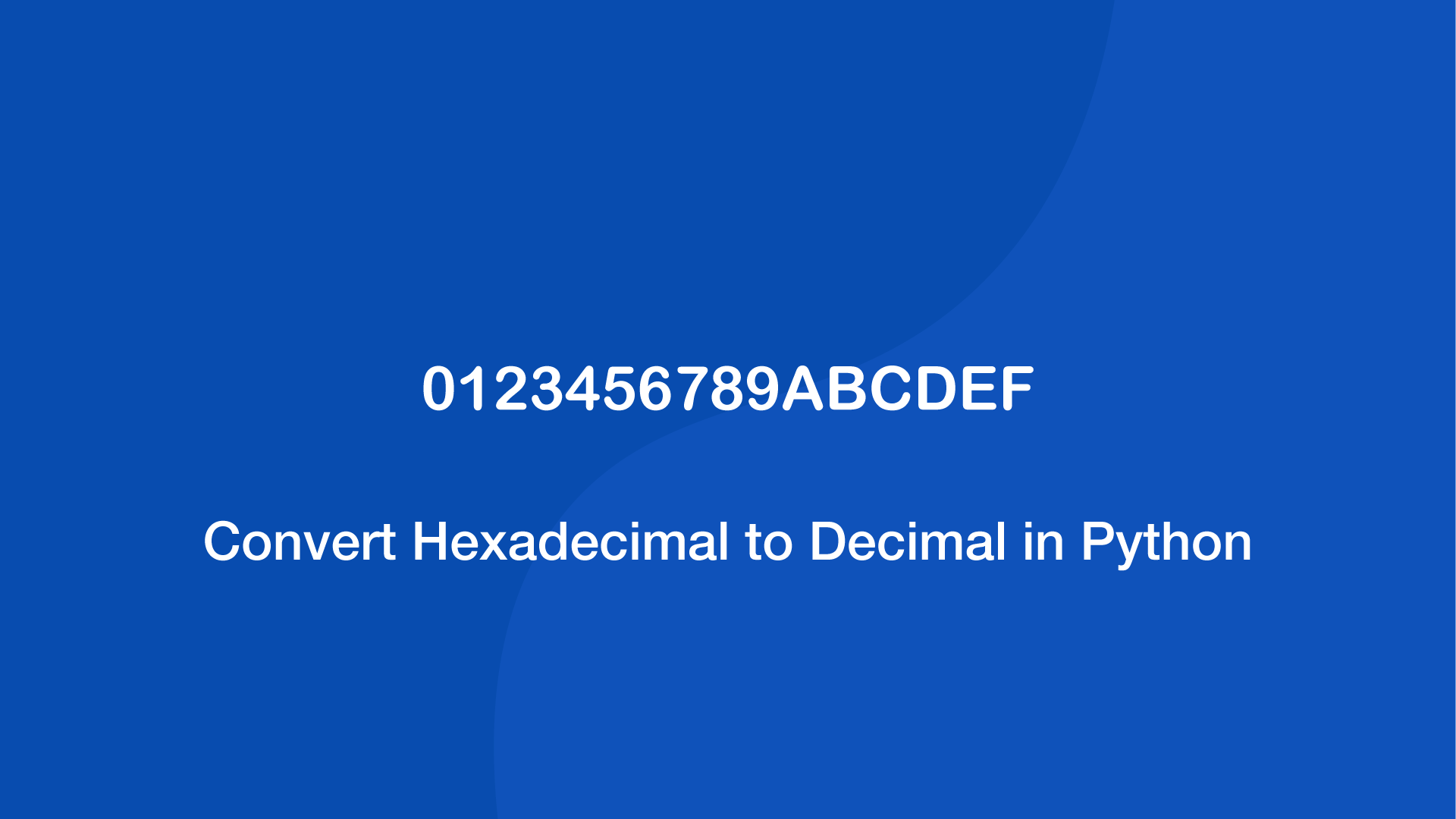# Convert Hexadecimal to Decimal in PythonTo convert a hexadecimal value to decimal in Python, use the built-in int() function. Pass the hex number as the first number and 16 as the second.

## Hex to Dec with the Python int() Function

To demonstrate this, let's change 8AB into its decimal representation using the int() function:

``````print(int('8AB', 16))
``````
``````2219
``````

The reason why we use 16 as the second argument is to let the function know that it is being handed a base 16 value to convert to a decimal.

## Using ast.literal_eval() to Convert Hexadecimal to Decimal in Python

Another way of converting hex values to decimals is with the `ast.literal_eval()` function. Here is an example of how to use it:

``````from ast import literal_eval

result = literal_eval('0x8AB')

print(result)
``````
``````2219
``````

Make sure the hex value is prefixed with 0x or the literal_eval() parser will throw an error.

#### Related Tutorials### How to Convert Hexadecimal to Binary in Python

November 22, 2021### How to Add Two Hex Numbers in Python

November 16, 2021### How to use the parseInt() Function in JavaScript

July 15, 2021### How to Convert a String to a Number in JavaScript

August 20, 2020### How to Convert a String to a Double in Python

April 28, 2021April 07, 2021